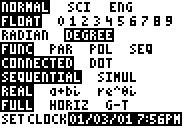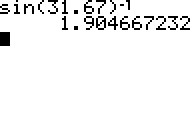# Knowledge Base

## Solution 25850: Calculating Secant, Cosecant and Cotangent Using the TI-83 Family, TI-84 Plus Family and TI-Nspire™ Handheld in TI-84 Plus Mode.

### How do I calculate secant, cosecant and cotangent using a TI-83 family, TI-84 Plus family and TI-Nspire handheld in TI-84 Plus mode?

The TI-83 family, TI-84 Plus family and TI-Nspire handheld in TI-84 Plus mode do not have built-in secant, cosecant and cotangent trigonometric functions. To compute these trigonometric functions, use the properties of trigonometric functions which are listed below.

cosecant(x) = csc(x) = sin(x)^-1

secant(x) = sec(x) = cos(x)^-1

cotangent(x) = cot(x) = tan(x)^-1

For Example: Solve for the cosecant of 31.67 degrees.

1) Be sure the calculator is set to Degree mode before beginning. To do this, press the [MODE] key, move the cursor to DEGREE and press [ENTER].